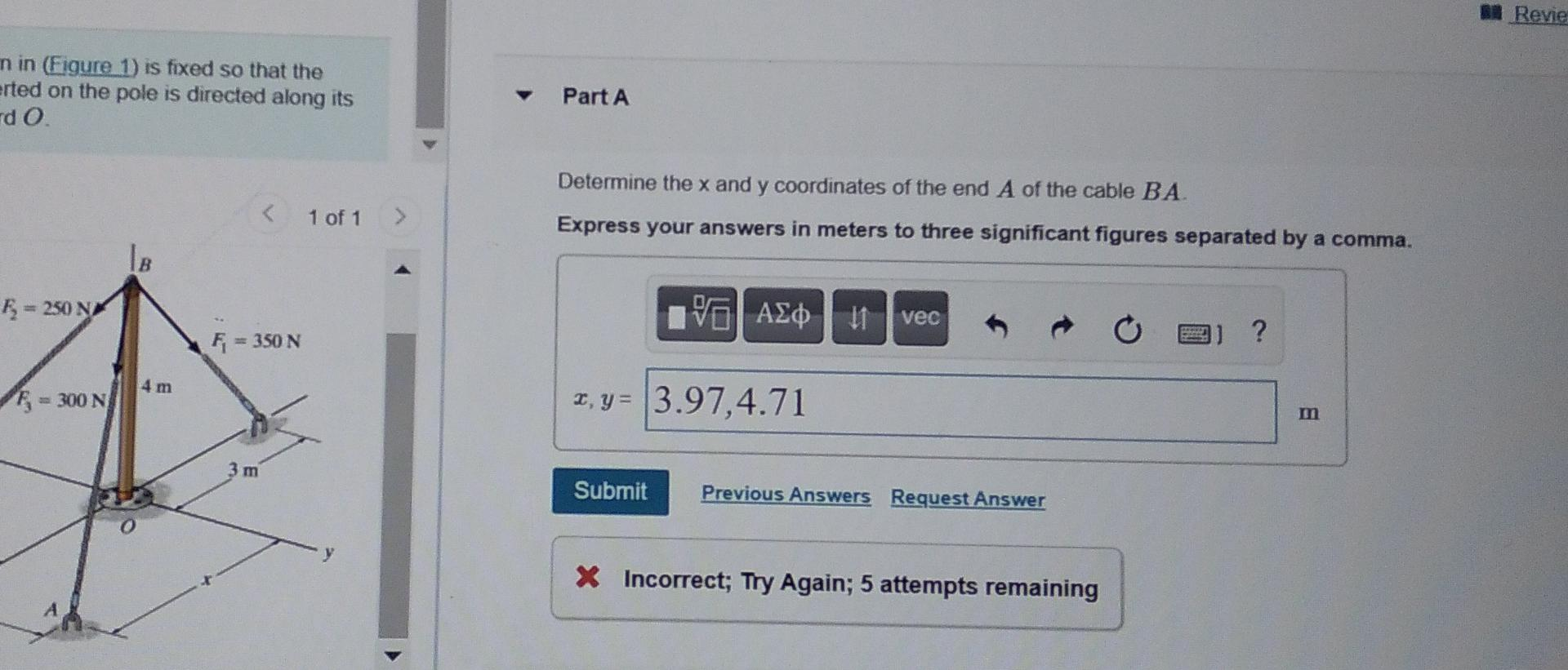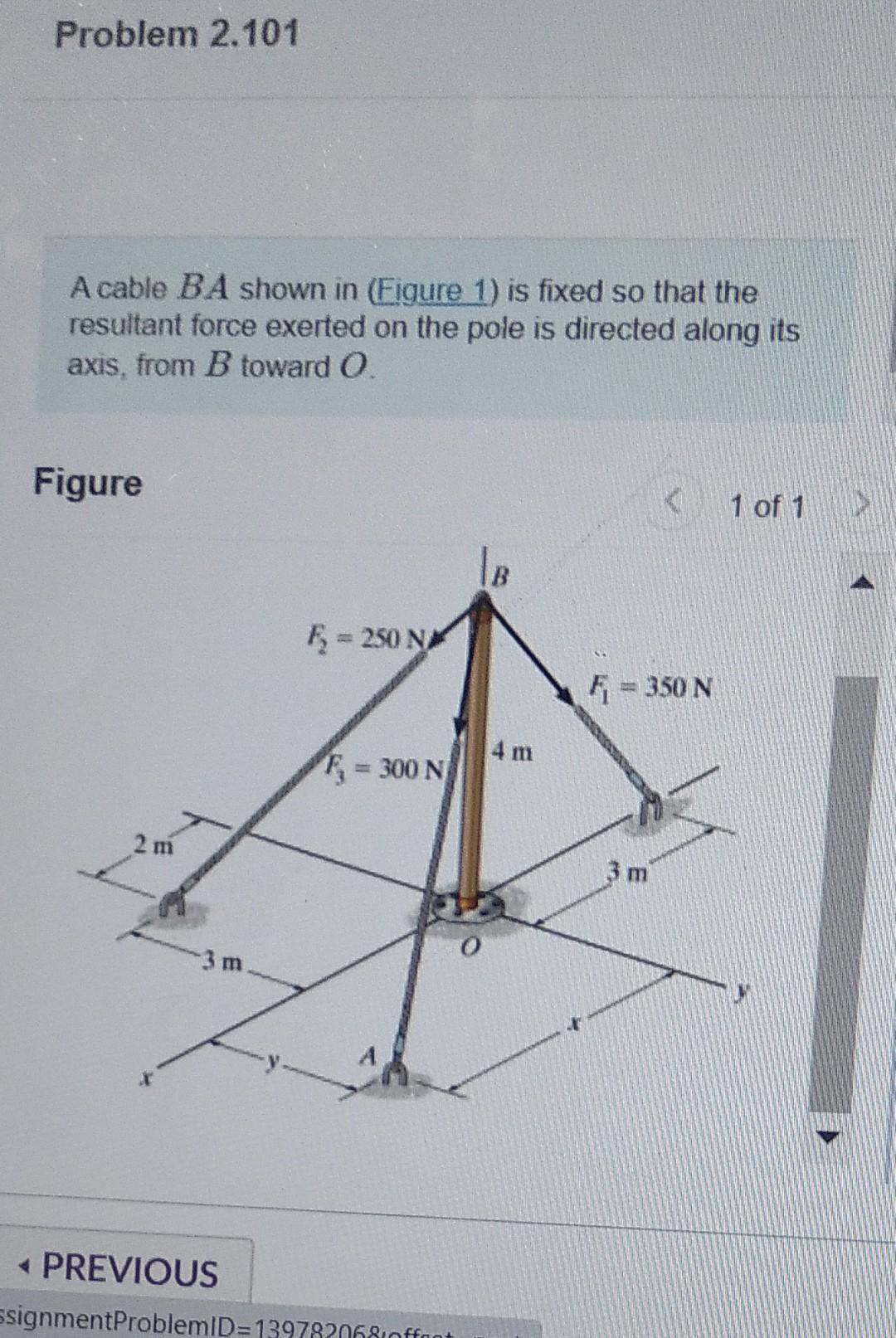# Question Solved1 AnswerRevie n in (Figure 1) is fixed so that the erted on the pole is directed along its do Part A Determine the x and y coordinates of the end A of the cable BA. 1 of 1 > Express your answers in meters to three significant figures separated by a comma. le E = 250 NM ΤΑ ΑΣΦ 11 vec ? Fi- = 350 N 4 m F = 300 N I, y = 3.97,4.71 m 3 m Submit Previous Answers Request Answer X Incorrect; Try Again; 5 attempts remaining Problem 2.101 A cable BA shown in (Figure 1) is fixed so that the resultant force exerted on the pole is directed along its axis, from B toward O. Figure 1 of 1 5 = 250 N = 350 N 4 m F = 300 N 2 m 3 m 3 m PREVIOUS ssignmentProblemID=139782068inffont4R95L9 The Asker · Mechanical EngineeringTranscribed Image Text: Revie n in (Figure 1) is fixed so that the erted on the pole is directed along its do Part A Determine the x and y coordinates of the end A of the cable BA. 1 of 1 > Express your answers in meters to three significant figures separated by a comma. le E = 250 NM ΤΑ ΑΣΦ 11 vec ? Fi- = 350 N 4 m F = 300 N I, y = 3.97,4.71 m 3 m Submit Previous Answers Request Answer X Incorrect; Try Again; 5 attempts remaining Problem 2.101 A cable BA shown in (Figure 1) is fixed so that the resultant force exerted on the pole is directed along its axis, from B toward O. Figure 1 of 1 5 = 250 N = 350 N 4 m F = 300 N 2 m 3 m 3 m PREVIOUS ssignmentProblemID=139782068inffont
More
Transcribed Image Text: Revie n in (Figure 1) is fixed so that the erted on the pole is directed along its do Part A Determine the x and y coordinates of the end A of the cable BA. 1 of 1 > Express your answers in meters to three significant figures separated by a comma. le E = 250 NM ΤΑ ΑΣΦ 11 vec ? Fi- = 350 N 4 m F = 300 N I, y = 3.97,4.71 m 3 m Submit Previous Answers Request Answer X Incorrect; Try Again; 5 attempts remaining Problem 2.101 A cable BA shown in (Figure 1) is fixed so that the resultant force exerted on the pole is directed along its axis, from B toward O. Figure 1 of 1 5 = 250 N = 350 N 4 m F = 300 N 2 m 3 m 3 m PREVIOUS ssignmentProblemID=139782068inffont